Ex 6.3

Chapter 6 Class 12 Application of Derivatives (Term 1)
Serial order wise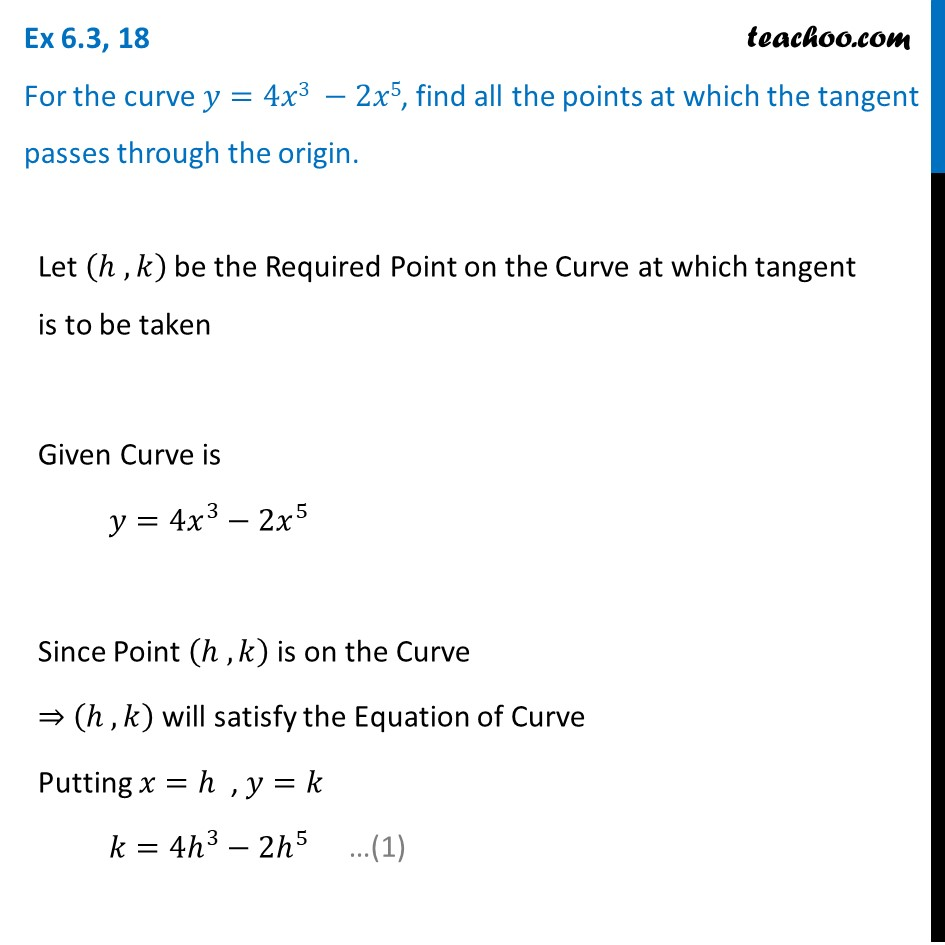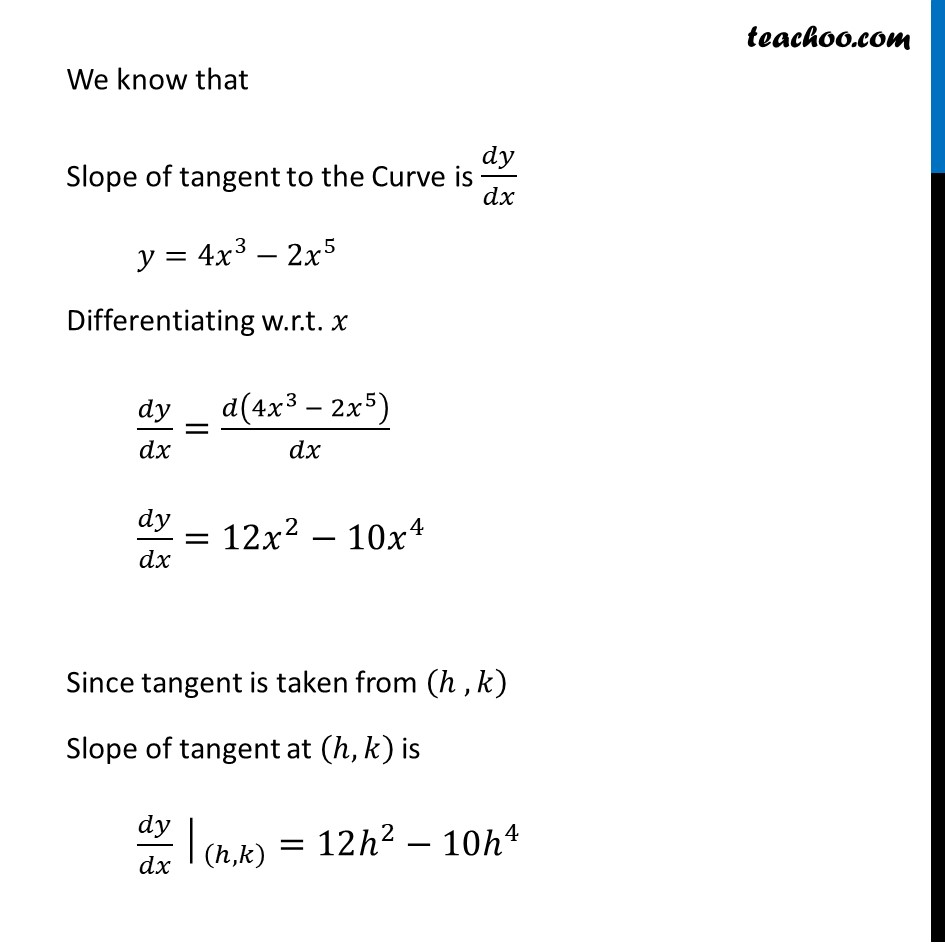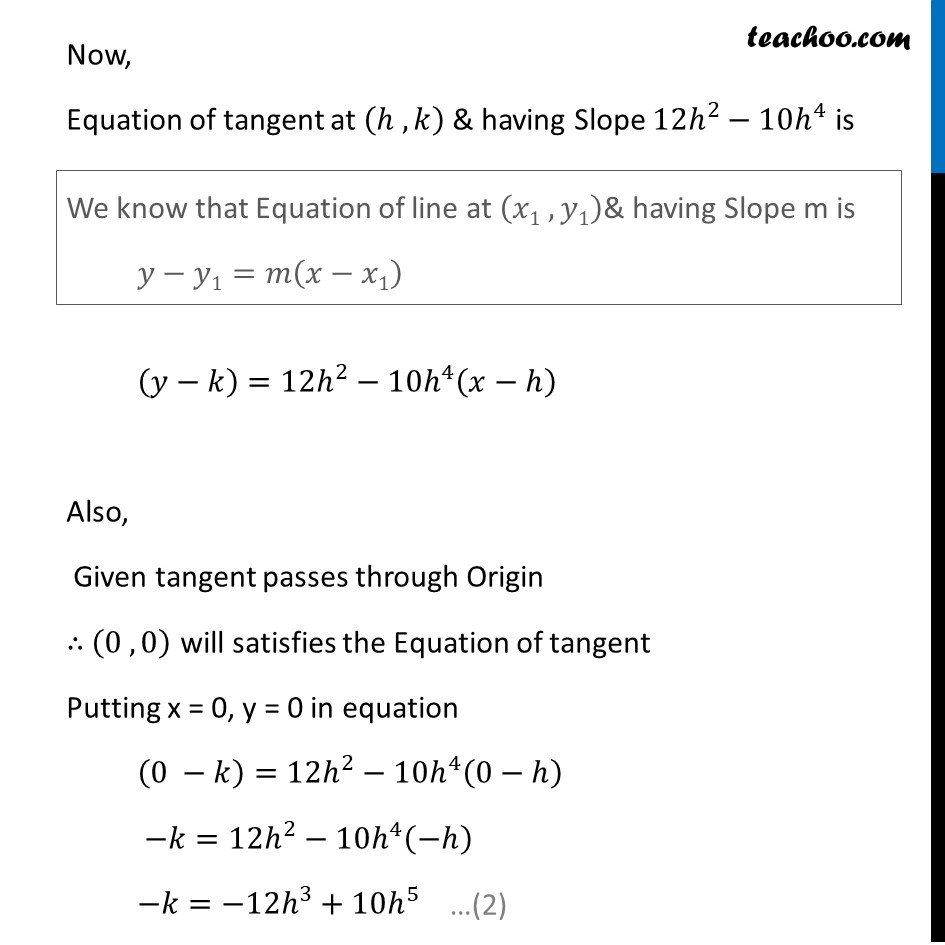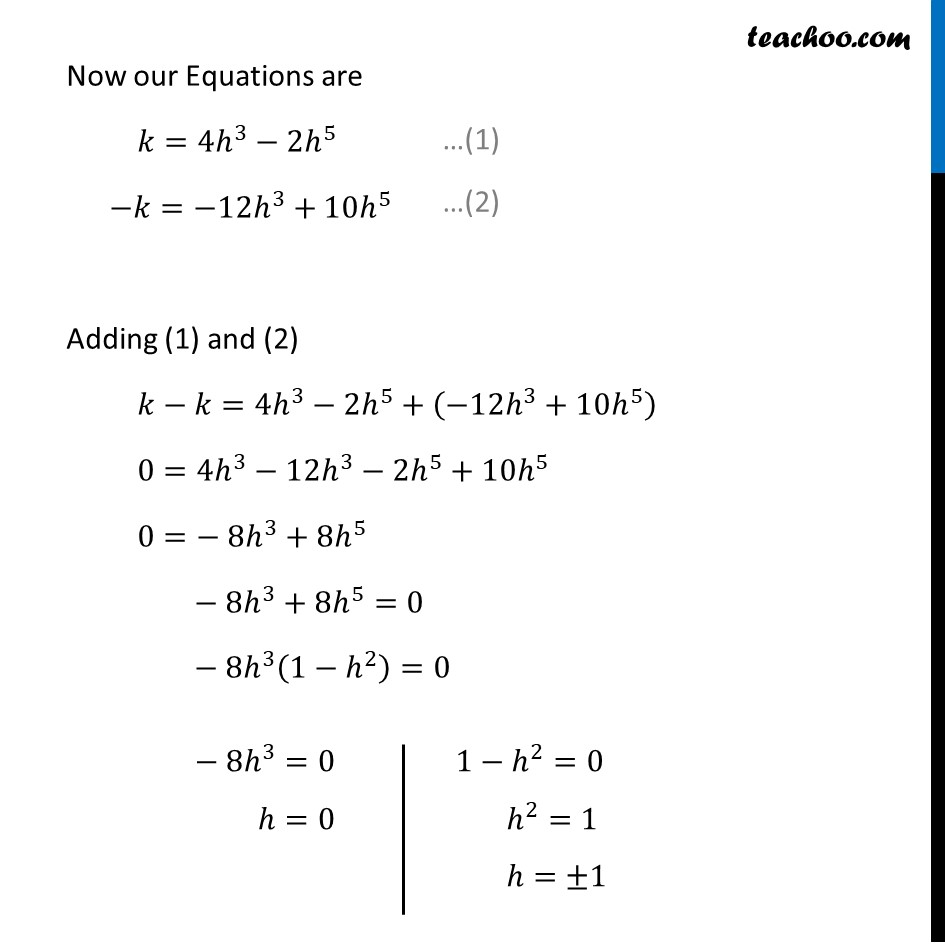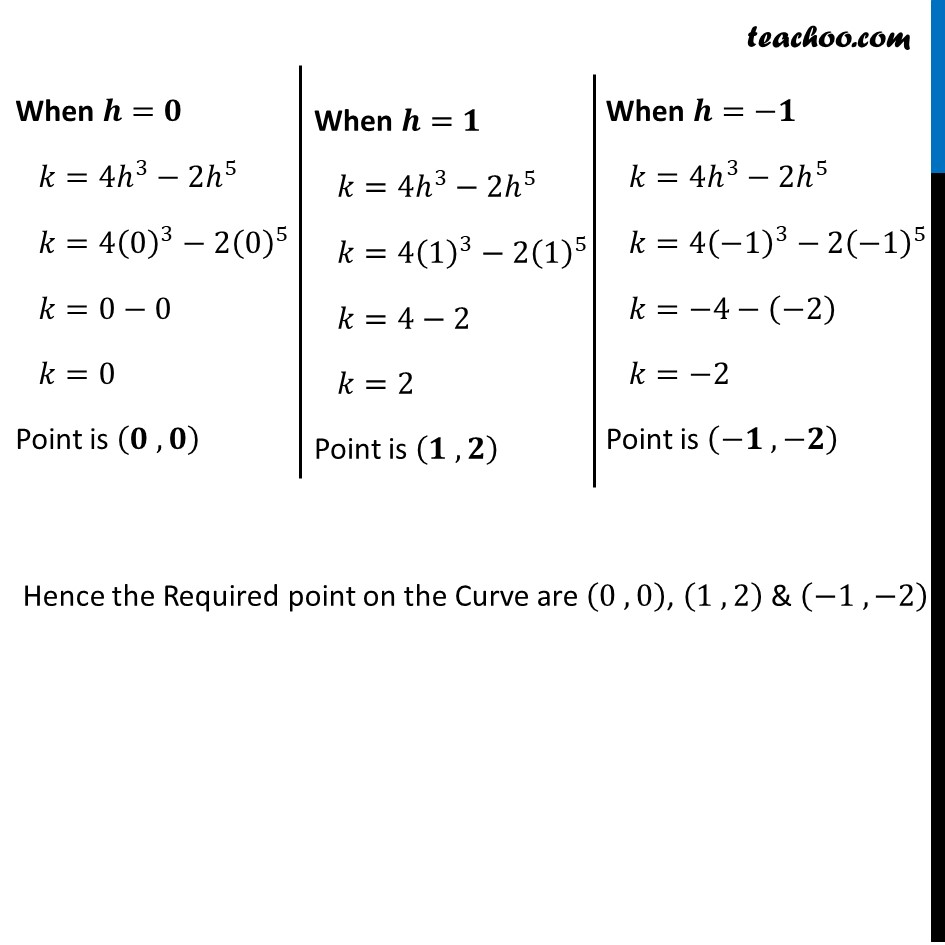### Transcript

Ex 6.3, 18 For the curve 𝑦=4𝑥3 −2𝑥5, find all the points at which the tangent passes through the origin.Let (ℎ , 𝑘) be the Required Point on the Curve at which tangent is to be taken Given Curve is 𝑦=4𝑥^3−2𝑥^5 Since Point (ℎ , 𝑘) is on the Curve ⇒ (ℎ , 𝑘) will satisfy the Equation of Curve Putting 𝑥=ℎ , 𝑦=𝑘 𝑘=4ℎ^3−2ℎ^5 We know that Slope of tangent to the Curve is 𝑑𝑦/𝑑𝑥 𝑦=4𝑥^3−2𝑥^5 Differentiating w.r.t. 𝑥 𝑑𝑦/𝑑𝑥=𝑑(4𝑥^(3 )− 2𝑥^5 )/𝑑𝑥 𝑑𝑦/𝑑𝑥=12𝑥^2−10𝑥^4 Since tangent is taken from (ℎ , 𝑘) Slope of tangent at (ℎ, 𝑘) is 〖𝑑𝑦/𝑑𝑥│〗_((ℎ, 𝑘) )=12ℎ^2−10ℎ^4 Now, Equation of tangent at (ℎ , 𝑘) & having Slope 12ℎ^2−10ℎ^4 is (𝑦−𝑘)=12ℎ^2−10ℎ^4 (𝑥−ℎ) Also, Given tangent passes through Origin ∴ (0 , 0) will satisfies the Equation of tangent Putting x = 0, y = 0 in equation (0 −𝑘)=12ℎ^2−10ℎ^4 (0−ℎ) −𝑘=12ℎ^2−10ℎ^4 (−ℎ) −𝑘=−12ℎ^3+10ℎ^5 We know that Equation of line at (𝑥1 , 𝑦1)& having Slope m is 𝑦−𝑦1=𝑚(𝑥−𝑥1) Now our Equations are 𝑘=4ℎ^3−2ℎ^5 −𝑘=−12ℎ^3+10ℎ^5 Adding (1) and (2) 𝑘−𝑘=4ℎ^3−2ℎ^5+(−12ℎ^3+10ℎ^5 ) 0=4ℎ^3−12ℎ^3−2ℎ^5+10ℎ^5 0=− 8ℎ^3+8ℎ^5 − 8ℎ^3+8ℎ^5=0 − 8ℎ^3 (1−ℎ^2 )=0 − 8ℎ^3=0 ℎ=0 1−ℎ^2=0 ℎ^2=1 ℎ=±1 When 𝒉=𝟎 𝑘=4ℎ^3−2ℎ^5 𝑘=4(0)^3−2(0)^5 𝑘=0−0 𝑘=0 Point is (𝟎 , 𝟎) When 𝒉=𝟏 𝑘=4ℎ^3−2ℎ^5 𝑘=4(1)^3−2(1)^5 𝑘=4−2 𝑘=2 Point is (𝟏 , 𝟐) When 𝒉=−𝟏 𝑘=4ℎ^3−2ℎ^5 𝑘=4(−1)^3−2(−1)^5 𝑘=−4−(−2) 𝑘=−2 Point is (−𝟏 , −𝟐) Hence the Required point on the Curve are (0 , 0), (1 , 2) & (−1 , −2)